# Kurzweil-Henstock integral

(diff) ← Older revision | Latest revision (diff) | Newer revision → (diff)

The Denjoy and Perron integrals (cf. Denjoy integral; Perron integral), which are equivalent and more general than the Lebesgue integral, were introduced in the second decade of the 20th century to invert the finite ordinary derivative. Much more recently, J. Kurzweil [a6], and independently R. Henstock [a2], made a simple modification to the Riemann integral and defined another integral that is equivalent to the Denjoy and Perron integrals. This integral is known as the gauge integral, the Riemann-complete integral, the generalized Riemann integral, or the Kurzweil–Henstock integral, also abbreviated to K–H integral. A further simple modification, by E.J. MacShane [a10], gave an integral that is equivalent to the Lebesgue integral.

A positive functionon a compact intervalis called a gauge, and with any such gauge one can associate a full covering ofby: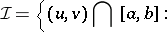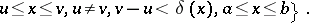This covering is said to be-fine. It is easy to see that every suchcan be used to give a partition of, also called-fine, that is, a partition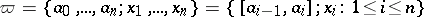, where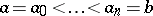,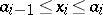,,. The existence of such a partition is known as Cousin's lemma, and is equivalent to the Bolzano–Weierstrass theorem. It is easy to see that there is no loss in generality in assuming the tags, the, to be end-points of the sub-intervals (that is, for alleitheror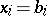), although it is not possible to assume the tags are always left, or right, end-points.

Once the existence of a-fine partition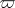is known, one can associate with any finite functionona Riemann sum,. The definition of the K–H integral follows that of the Riemann integral:is K–H-integrable onif there is a real numbersuch that for allthere exists a gaugesuch that for all-fine partitionsone has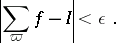(a1)

The numberis then the value of the integral of. The similarity of this definition to the classical Riemann definition means that the proofs of all the basic properties of the K–H integral follow those of the more elementary integral, corresponding to the case where the gauge is a continuous or constant function.

However, the power given by the use of a locally fine gauge, that can be made to adapt to the function better than a uniform gauge, is shown by the following two examples.

A) Letbe the derivative ofand define a gaugeforas follows: for allthere is a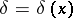such that. Ifis a-fine partition whose tags are always end-points, then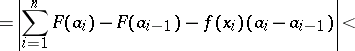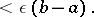So, an arbitrary finite derivative, is K–H-integrable.

B) Letbe a set of measure zero witharbitrary onbut zero elsewhere. Given an, let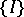be an open covering ofof total length less than. Define a gaugeas follows: ifthen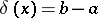, and if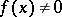then, whereis any interval from the open covering that contains. Ifis a-fine partition, then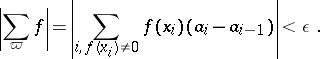Hence such anis K–H-integrable with integral value zero. This allows one: 1) to deduce that if two functions are equal almost-everywhere, then they are K–H-integrable together, and 2) to extend the definition of the integral to include functions that are only defined almost-everywhere.

Deeper properties, such as the continuity and differentiability properties of the primitive, a monotone convergence theorem, a proof that the absolutely integrable functions are precisely the Lebesgue-integrable functions, depend on the so-called Saks–Henstock lemma, [a1], [a2], [a3]: Ifis K–H-integrable, with indefinite integral, where, if,are as in the definition (a1) above, and if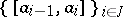is any collection of sub-intervals from the partition, then: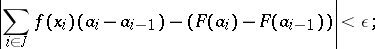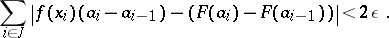As a simple application, suppose that the primitive of a K–H-integrable function is everywhere zero. Then one can show that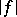is K–H-integrable to zero. Indeed, let,be as in the definition (a1) and let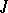be as in the Saks–Henstock lemma. Then the hypothesis and the lemma imply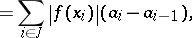which proves the statement.

There has been much work on these techniques, and they have been used to obtain many properties of the Denjoy and Perron integrals that were often proved by much more onerous methods. In particular, P.-Y. Lee has developed a theory of controlled convergence, [a8]. This theory gives to this integral a real convergence theorem, since once use can be made of the monotone convergence theorem one is really in the Lebesgue theory. His result is based on a Dzharsheishvili theorem that generalizes Vitali's theorem to the Denjoy integral.

The definition above can easily be extended to give a Stieltjes integral that is equivalent to Ward's Perron–Stieltjes integral, and even more general interval functions can be integrated very simply by this method, as has been shown in detail by R. Henstock, [a2], [a3], [a7].

If, in the definition of the-fine covering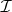the requirement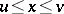is changed to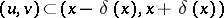, the above procedure will give the MacShane integral, which is equivalent to the Lebesgue integral. Inspection of A) shows that one needs the original form of the definition here. Further extensions ofhave been studied, such as requiring that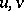belong to a set of densityat, or that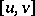be symmetric with respect to the tag, [a1], [a3], [a13]. The purpose of these extensions is to define integrals that will invert approximate and symmetric derivatives. Such extensions are far from obvious and require deep and subtle considerations. The same is true of the extensions of the theory that have been made to higher dimensions [a12]. However, these extensions are much more successful than the attempts to do the same for the Denjoy and Perron integrals. On the other hand, the extension of the Kurzweil–Henstock theory to general spaces is much less natural than the general measure theory that follows from the Lebesgue theory, [a5].

How to Cite This Entry:
Kurzweil-Henstock integral. Encyclopedia of Mathematics. URL: http://encyclopediaofmath.org/index.php?title=Kurzweil-Henstock_integral&oldid=14260
This article was adapted from an original article by P. Bullen (originator), which appeared in Encyclopedia of Mathematics - ISBN 1402006098. See original article